# VLOOKUP & HLOOKUP Combined – Excel & Google Sheets

This tutorial will demonstrate how to use the VLOOKUP and HLOOKUP Functions together to lookup a value in Excel and Google Sheets.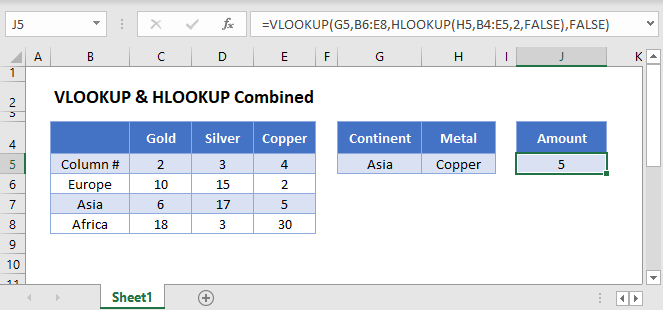## Combine VLOOKUP and HLOOKUP

Sometimes you need the column reference of your VLOOKUP formula to be dynamic. Usually, the best way to do this is by combining the VLOOKUP & MATCH Functions, but in this tutorial we will demonstrate the VLOOKUP-HLOOKUP combination instead.

``=VLOOKUP(G3,B4:E6,HLOOKUP(H3,B2:E3,2,FALSE),FALSE)``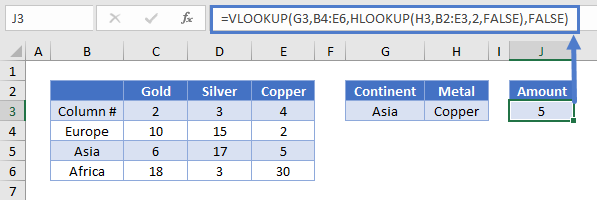In this example, our formula returns the amount of Copper in Asia, given the continent and the metal.

Let’s see how to combine these two popular functions.

### HLOOKUP Function

First add a helper row (Row 3 below) with the column numbers. Then use the HLOOKUP Function to return the column number from that row for use in the VLOOKUP Function.

``=HLOOKUP("Copper",B2:E3,2,FALSE)``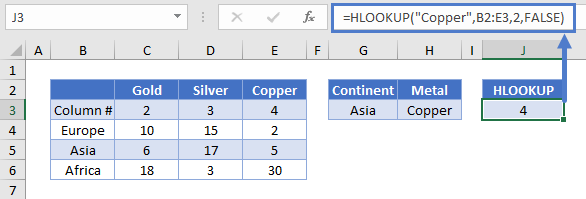### VLOOKUP Function

Once you have the column number, you can use it in your VLOOKUP formula as the col_index_num input.

``=VLOOKUP(lookup_value, table_array, col_index_num, [range_lookup])``
``=VLOOKUP(G3,B4:E6,J3,FALSE)``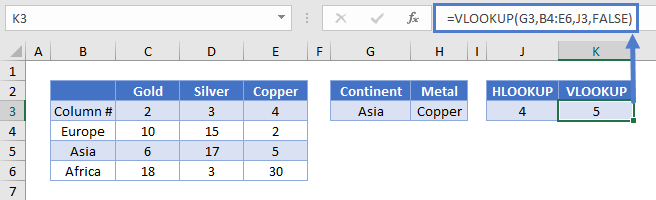Putting these formulas together, yields our original formula.

## VLOOKUP & HLOOKUP Combined in Google Sheets

All the examples explained above work the same in Google sheets as they do in Excel.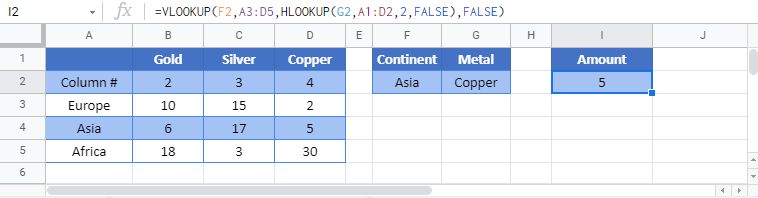### Excel Practice Worksheet

Practice Excel functions and formulas with our 100% free practice worksheets!